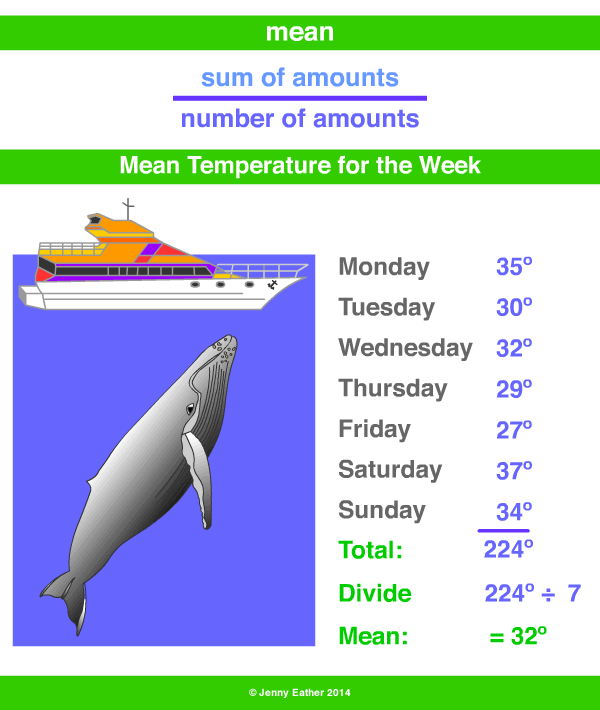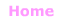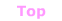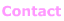Aa Bb Cc Dd Ee Ff Gg Hh Ii Jj Kk Ll Mm Nn Oo Pp Qq Rr Ss Tt Uu Vv Ww Xx Yy Zz

Mm

mean

• usually called the average and may be called the arithmetic mean.
• the mean is the total of all the scores or amounts,
divided by, how many scores or amounts there were.

how to work out the mean

1. Add up all the amounts.
2. Divide your total by the number of amounts.

EXAMPLE:© Jenny Eather 2014. All rights reserved.